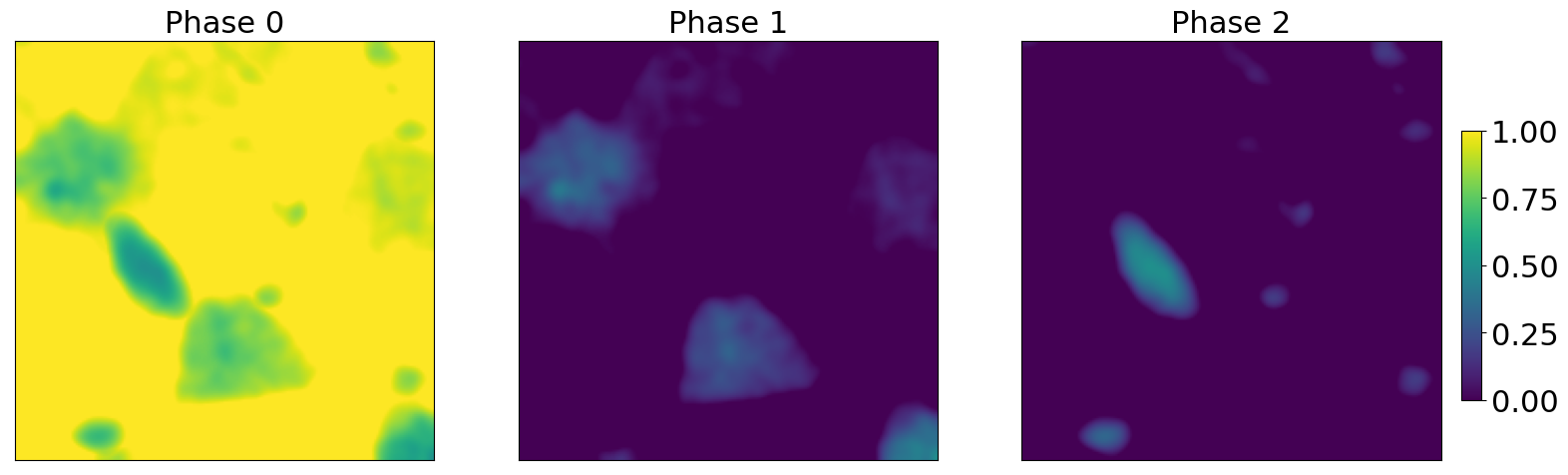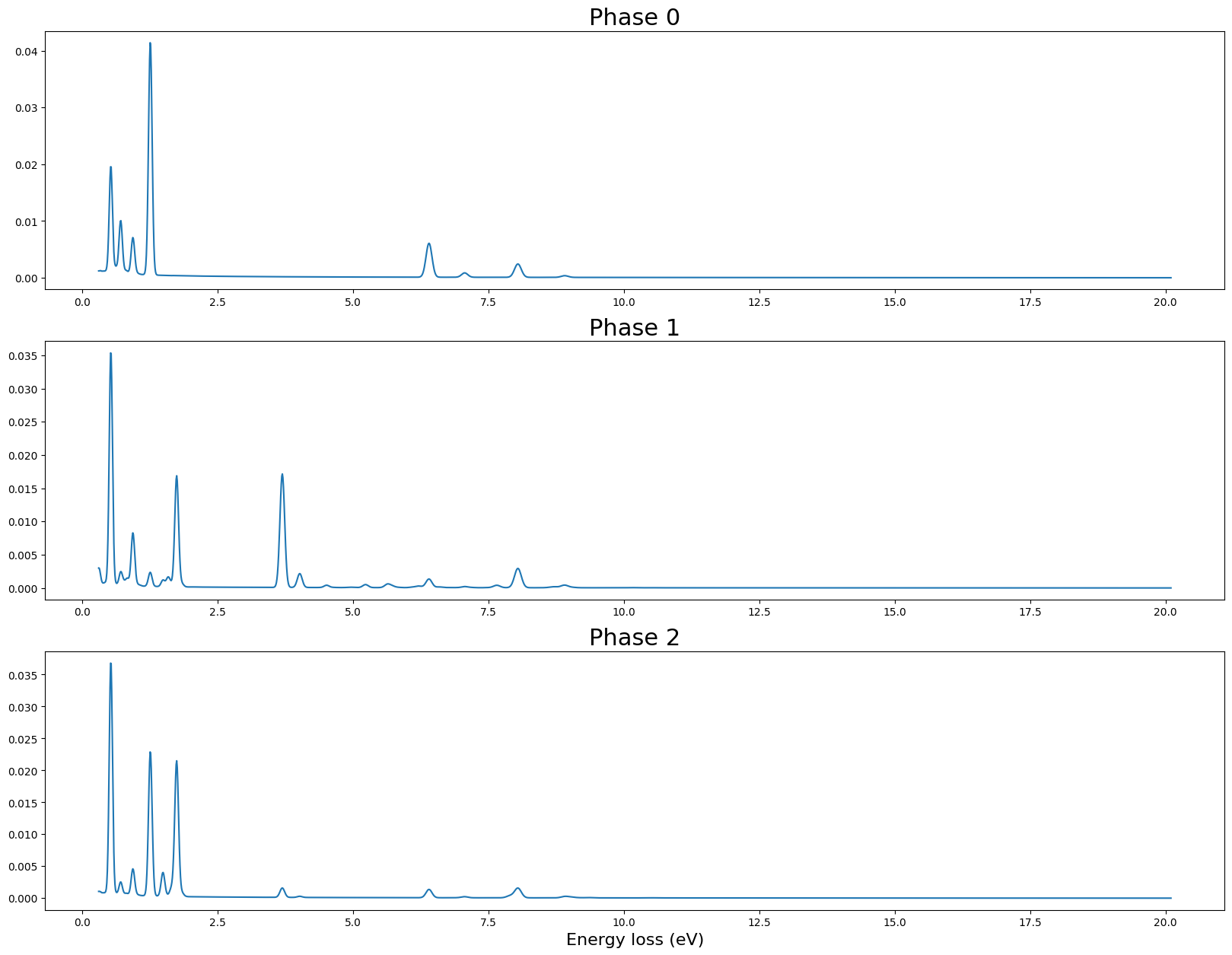# Generate simulated data

In this notebook we generate a simulated dataset based on experimental data. - The maps are directly extracted from the experimental data. - The phases (or spectra) are built using chemical composition extracted from the literature (citations to be included)

The output of this notebook is put in the generated_datasets

## Imports

:

%load_ext autoreload
%matplotlib inline

# espm imports
from espm.datasets.base import generate_dataset
from espm.weights.generate_weights import chemical_map_weights
from espm.models.generate_EDXS_phases import generate_modular_phases
from espm.models.EDXS_function import elts_list_from_dict_list

# Generic imports
from pathlib import Path
import matplotlib.pyplot as plt
import numpy as np
import hyperspy.api as hs


## Generate weights

We use an experimental dataset to create realistic abundance maps. Theses maps are based from chemical mapping of the dataset.

:

def get_repo_path():
"""Get the path to the git repository.

This is a bit of a hack, but it works.
"""
this_path = Path.cwd() / Path("generate_data.ipynb")
return this_path.resolve().parent.parent

# Path of the experimental dataset
path = get_repo_path() / Path("generated_datasets/71GPa_experimental_data.hspy")

# Creation of the weights
weights = chemical_map_weights( file = path, line_list = ["Fe_Ka","Ca_Ka"], conc_list = [0.5,0.5])

/home/docs/checkouts/readthedocs.org/user_builds/espm/envs/latest/lib/python3.11/site-packages/hyperspy/misc/utils.py:471: VisibleDeprecationWarning: Use of the binned attribute in metadata is going to be deprecated in v2.0. Set the axis.is_binned attribute instead.
warnings.warn(
/home/docs/checkouts/readthedocs.org/user_builds/espm/envs/latest/lib/python3.11/site-packages/hyperspy/misc/utils.py:471: VisibleDeprecationWarning: Use of the binned attribute in metadata is going to be deprecated in v2.0. Set the axis.is_binned attribute instead.
warnings.warn(


### Plot the results

:

fig,axs = plt.subplots(1,3,figsize = (20,20*3))
for j in range(axs.shape) :
im = axs[j].imshow(weights[:,:,j],vmin = 0, vmax = 1.0)
axs[j].tick_params(axis = "both",width = 0,labelbottom = False,labelleft = False)
axs[j].set_title("Phase {}".format(j),fontsize = 22)

cbar_ax = fig.add_axes([0.85, 0.47, 0.01, 0.045])
fig.colorbar(im,cax=cbar_ax)
cbar_ax.tick_params(labelsize=22)## Generate phases

We generate phases based on values extracted from the literature. The phases we try to simulate here are Ferropericlase, Bridgmanite and Ca-perovskite.

We use dictionnaries to input the modelling parameters.

:

# Elemental concetration of each phase
elts_dicts = [
# Pseudo ferropericlase
{
"Mg" : 0.522, "Fe" : 0.104, "O" : 0.374, "Cu" : 0.05
},
# Pseudo Ca-Perovskite
{
"Mg" : 0.020, "Fe" : 0.018, "Ca" : 0.188, "Si" : 0.173, "Al" : 0.010, "O" : 0.572, "Ti" : 0.004, "Cu" : 0.05, "Sm" : 0.007, "Lu" : 0.006, "Nd" : 0.006
},
# Pseudo Bridgmanite
{
"Mg" : 0.445, "Fe" : 0.035, "Ca" : 0.031, "Si" : 0.419, "Al" : 0.074, "O" : 1.136, "Cu" : 0.05, "Hf" : 0.01
}]

# Parameters of the bremsstrahlung
brstlg_pars = [
{"b0" : 0.0001629, "b1" : 0.0009812},
{"b0" : 0.0007853, "b1" : 0.0003658},
{"b0" : 0.0003458, "b1" : 0.0006268}
]

# Model parameters : energy scale, detector broadening, x-ray emission database, beam energy, absorption parameters, detector efficiency
model_params = {
"e_offset" : 0.3,
"e_size" : 1980,
"e_scale" : 0.01,
"width_slope" : 0.01,
"width_intercept" : 0.065,
"db_name" : "default_xrays.json",
"E0" : 200,
"params_dict" : {
"Abs" : {
"thickness" : 100.0e-7,
"toa" : 35,
"density" : 4.5,
"atomic_fraction" : False
},
"Det" : "SDD_efficiency.txt"
}
}

# miscellaneous paramaters : average detected number of X-rays per pixel, phases densities, output folder, model name, random seed
data_dict = {
"N" : 100,
"densities" : [1.2,1.0,0.8],
"data_folder" : "71GPa_synthetic_N100",
"model" : "EDXS",
"seed" : 42
}

:

phases = generate_modular_phases(
elts_dicts=elts_dicts, brstlg_pars = brstlg_pars,
scales = [1, 1, 1],
model_params= model_params,
seed = 42
)
# scales : bremsstrahlung parameters modifiers

elements = elts_list_from_dict_list(elts_dicts)


### Plot the results

:

fig,axs = plt.subplots(3,1,figsize = (20,15))

# Build the energy scale
x = np.linspace(
model_params["e_offset"],
model_params["e_offset"]+model_params["e_scale"]*model_params["e_size"],
num=model_params["e_size"])

for j in range(axs.shape) :
axs[j].plot(x,phases[j])
axs[j].set_title("Phase {}".format(j),fontsize = 22)

axs[-1].set_xlabel("Energy loss (eV)",fontsize = 16)

:

Text(0.5, 0, 'Energy loss (eV)')#### Generate the data

It will produce 1 spectrum images/sample in the target folder.

You can replace seed_range by the number of samples you want to generate.

:

generate_dataset( phases = phases,
weights = weights,
model_params = model_params,
misc_params = data_dict,
base_seed=data_dict["seed"],
sample_number=1,
elements = elements)

100%|██████████| 1/1 [00:07<00:00,  7.43s/it]


The previous command will save the data in the generated_datasets folder defined in the espm.conf.py file.

You can also define the path where the data will be saved using the “base_path” argument